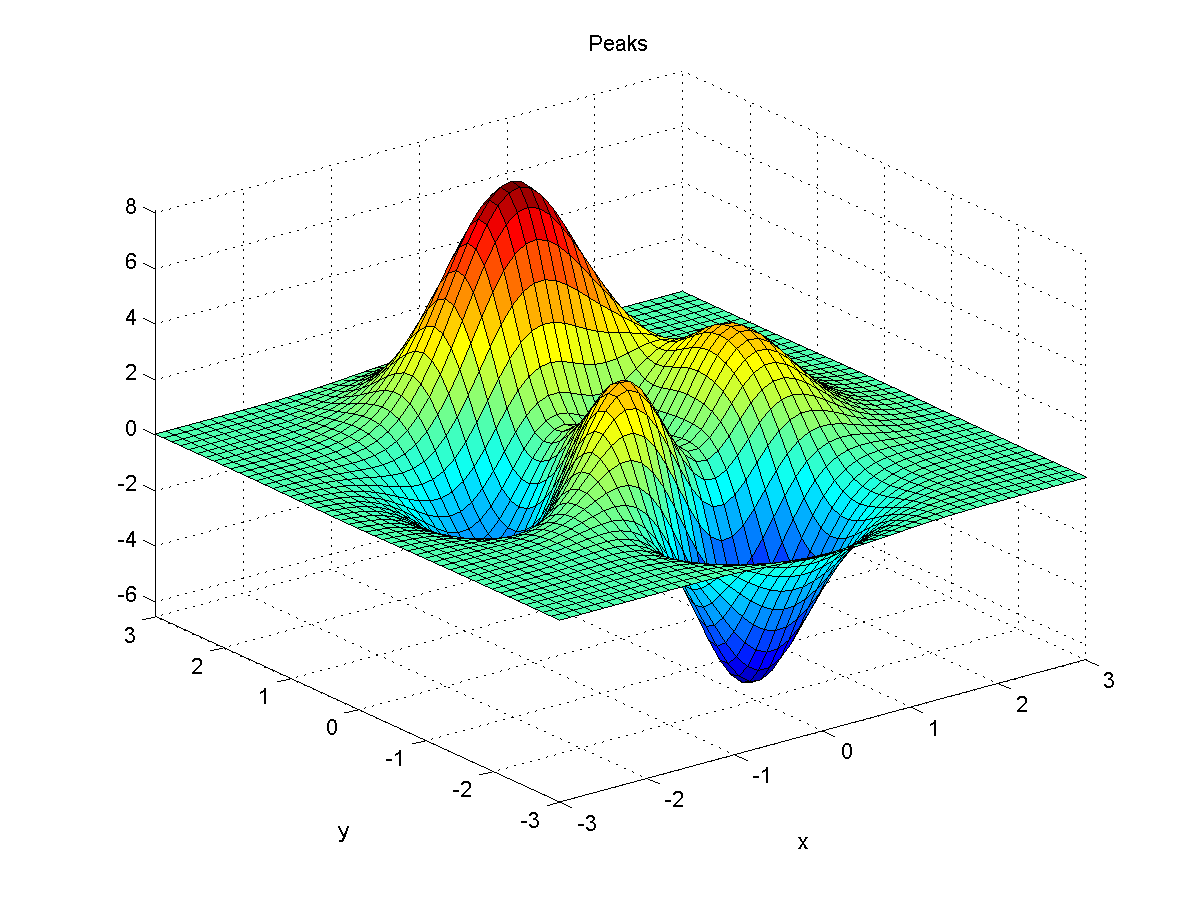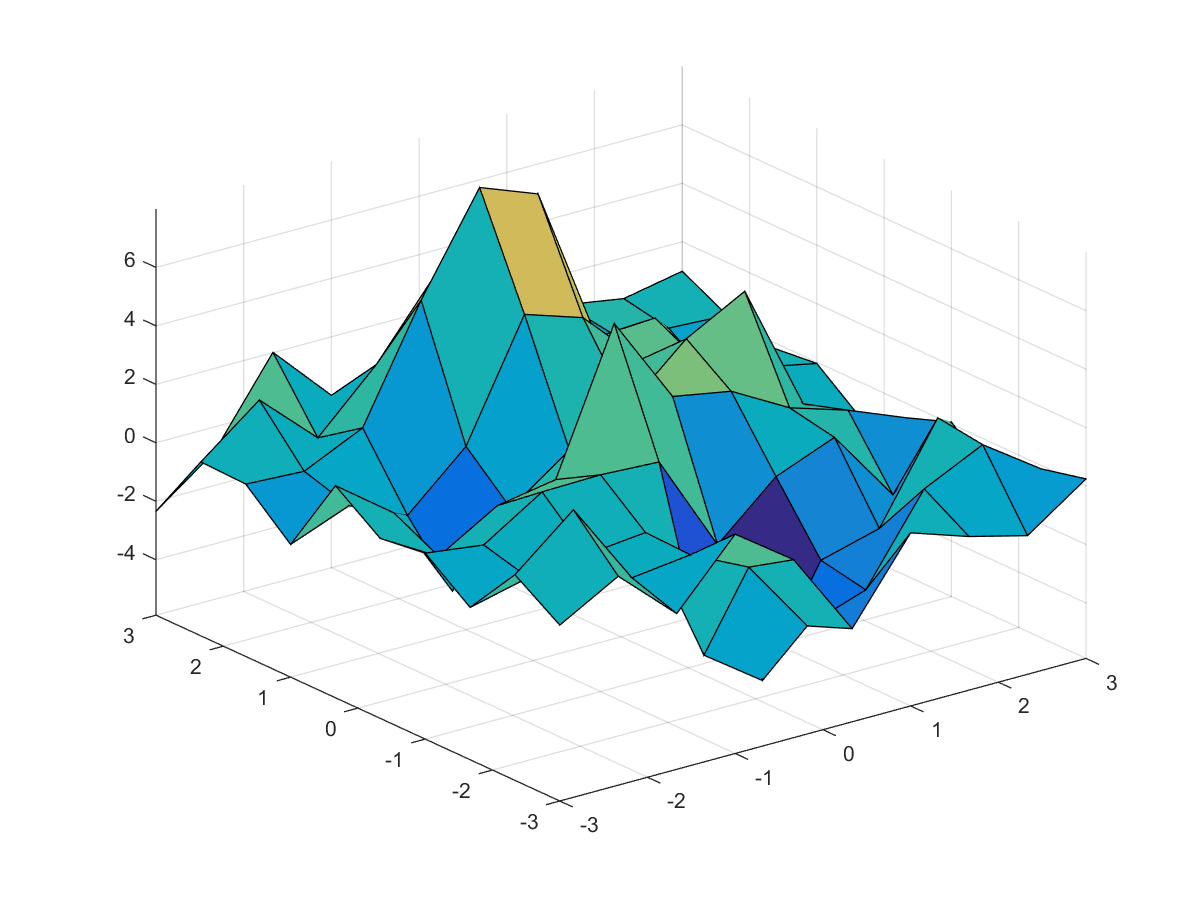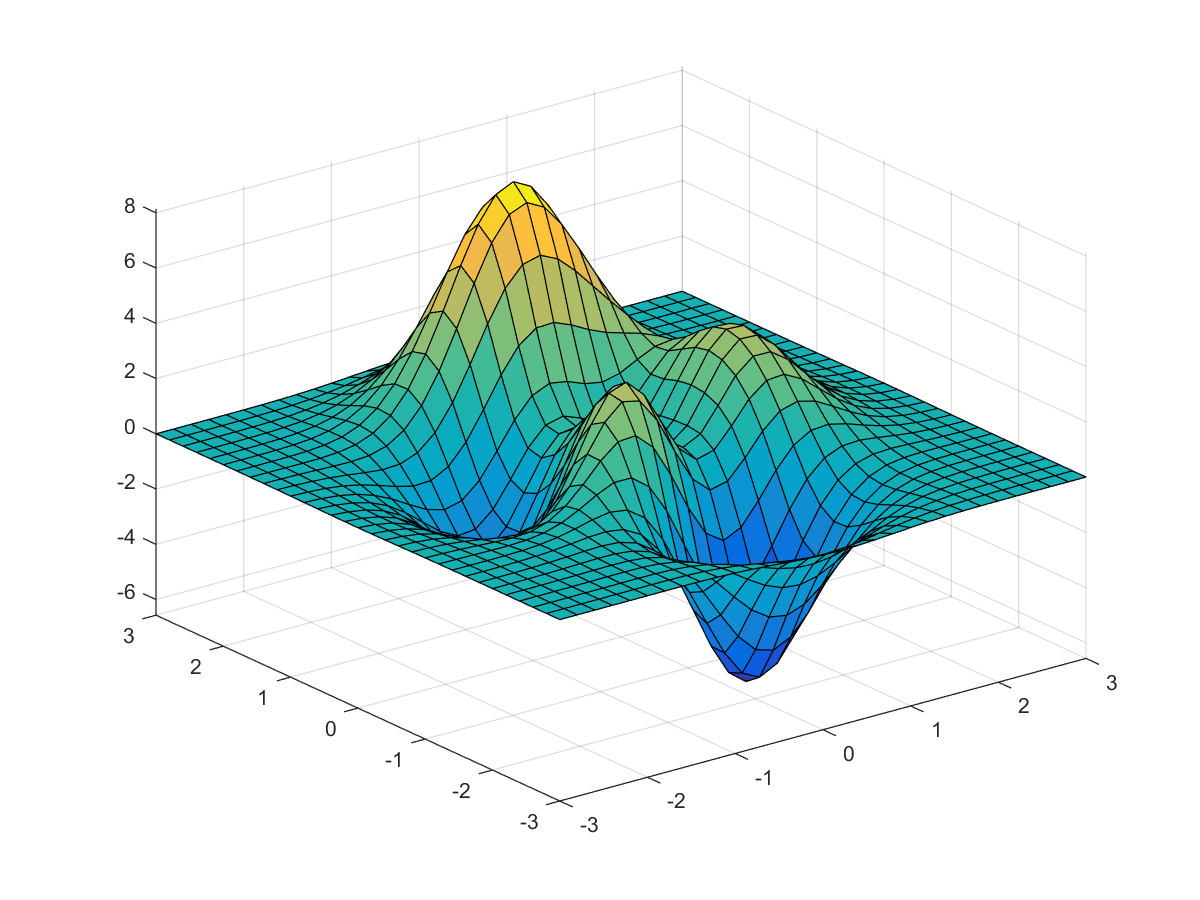## 10-2 嚙踝蕭嚙踝蕭嚙踝蕭嚙踝蕭嚙踝蕭嚙踝蕭嚙踝蕭嚙踝蕭嚙踝蕭嚙踝蕭嚙踝蕭嚙踝蕭嚙踝蕭嚙?

$$y = f(\mathbf{x}) = \theta_1f_1(\mathbf{x}) + \theta_2f_2(\mathbf{x}) + \cdots + \theta_nf_n(\mathbf{x})$$

$$\underbrace{ \left[ \begin{matrix} f_1(\mathbf{x}_1) & \cdots & f_n(\mathbf{x}_1) \\ f_1(\mathbf{x}_2) & \cdots & f_n(\mathbf{x}_2) \\ \vdots & \vdots & \vdots\\ f_1(\mathbf{x}_m) & \cdots & f_n(\mathbf{x}_m) \\ \end{matrix} \right] }_\mathbf{A} \underbrace{ \left[ \begin{matrix} \theta_1\\ \vdots\\ \theta_n\\ \end{matrix} \right] }_\mathbf{\theta} = \underbrace{ \left[ \begin{matrix} y_1\\ y_2\\ \vdots\\ y_m\\ \end{matrix} \right] }_\mathbf{y}$$

$$E(\mathbf{\theta})=\|\mathbf{e}\|^2=\mathbf{e}^T\mathbf{e}= (\mathbf{A}\mathbf{\theta}-\mathbf{y})^T (\mathbf{A}\mathbf{\theta}-\mathbf{y})$$

Hint$$z = 3(1-x)^2 e^{-x^2-(y+1)^2}-10\left(\frac{x}{5}-x^3-y^5\right) e^{-x^2-y^2}- \frac{1}{3} e^{-(x+1)^2-y^2}$$

• 數學模型的基底函數已知
• 訓練資料包含正規分佈的雜訊

$$\begin{array}{rcl} z & = & 3(1-x)^2 e^{-x^2-(y+1)^2}-10\left(\frac{x}{5}-x^3-y^5\right) e^{-x^2-y^2}- \frac{1}{3} e^{-(x+1)^2-y^2} + noise\\ & = & 3 f_1(x, y) - 10 f_2(x, y) - \frac{1}{3} f_3(x, y) + noise\\ & = & \theta_1 f_1(x, y) + \theta_2 f_2(x, y) + \theta_3 f_3(x, y) + noise\\ \end{array}$$

Example 1: 10-曲線擬合與迴歸分析/peaks01.mpointNum = 10; [xx, yy, zz] = peaks(pointNum); zz = zz + randn(size(zz)); % 加入雜訊 surf(xx, yy, zz); axis tightExample 2: 10-曲線擬合與迴歸分析/peaks02.mpointNum = 10; [xx, yy, zz] = peaks(pointNum); zz = zz + randn(size(zz))/10; % 加入雜訊 x = xx(:); % 轉為行向量 y = yy(:); % 轉為行向量 z = zz(:); % 轉為行向量 A = [(1-x).^2.*exp(-(x.^2)-(y+1).^2), (x/5-x.^3-y.^5).*exp(-x.^2-y.^2), exp(-(x+1).^2-y.^2)]; theta = A\z % 最佳的 theta 值 theta = 3.0392 -10.0407 -0.4093

Example 3: 10-曲線擬合與迴歸分析/peaks03.mpointNum = 10; [xx, yy, zz] = peaks(pointNum); zz = zz + randn(size(zz))/10; % 加入雜訊 x = xx(:); y = yy(:); z = zz(:); % 轉為行向量 A = [(1-x).^2.*exp(-(x.^2)-(y+1).^2), (x/5-x.^3-y.^5).*exp(-x.^2-y.^2), exp(-(x+1).^2-y.^2)]; theta = A\z; % 最佳的 theta 值 % 畫出預測的曲面 pointNum = 31; [xx, yy] = meshgrid(linspace(-3, 3, pointNum), linspace(-3, 3, pointNum)); x = xx(:); y = yy(:); % 轉為行向量 A = [(1-x).^2.*exp(-(x.^2)-(y+1).^2), (x/5-x.^3-y.^5).*exp(-x.^2-y.^2), exp(-(x+1).^2-y.^2)]; zz = reshape(A*theta, pointNum, pointNum); surf(xx, yy, zz); axis tightMATLAB程式設計：進階篇